## Operations with Square Roots

You can perform a number of different operations with square roots. Some of these operations involve a single radical sign, while others can involve many radical signs. The rules governing these operations should be carefully reviewed.

#### Under a single radical sign

You may perform operations under a single radical sign.

##### Example 1

Perform the operation indicated.

1.2.3.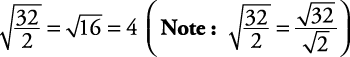4.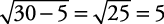5.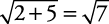#### When radical values are alike

You can add or subtract square roots themselves only if the values under the radical sign are equal. Then simply add or subtract the coefficients (numbers in front of the radical sign) and keep the original number in the radical sign.

##### Example 2

Perform the operation indicated.

1.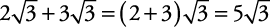2.3.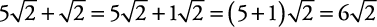Note that the coefficient 1 is understood in.

#### When radical values are different

You may not add or subtract different square roots.

##### Example 3

1.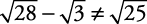2.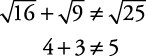#### Addition and subtraction of square roots after simplifying

Sometimes, after simplifying the square root(s), addition or subtraction becomes possible. Always simplify if possible.

##### Example 4

1.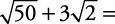These cannot be added until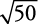is simplified.Now, because both are alike under the radical sign,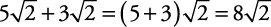2.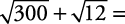Try to simplify each one.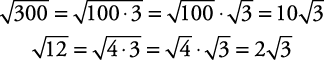Now, because both are alike under the radical sign,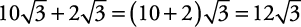#### Products of nonnegative roots

Remember that in multiplication of roots, the multiplication sign may be omitted. Always simplify the answer when possible.

##### Example 5

Multiply.

1.2. If each variable is nonnegative,3. If each variable is nonnegative,4. If each variable is nonnegative,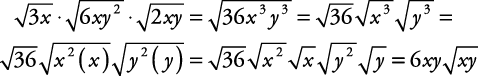5.#### Quotients of nonnegative roots

For all positive numbers,In the following examples, all variables are assumed to be positive.

##### Example 6

Divide. Leave all fractions with rational denominators.

1.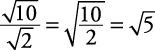2.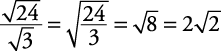3.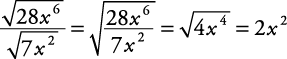4.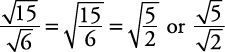Note that the denominator of this fraction in part (d) is irrational. In order to rationalize the denominator of this fraction, multiply it by 1 in the form of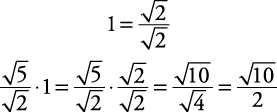##### Example 7

Divide. Leave all fractions with rational denominators.

1.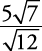2. First simplify: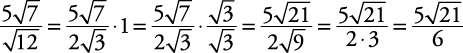or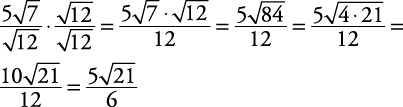3.4.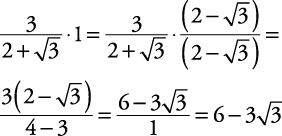Note: In order to leave a rational term in the denominator, it is necessary to multiply both the numerator and denominator by the conjugate of the denominator. The conjugate of a binomial contains the same terms but the opposite sign. Thus, ( x + y) and ( xy) are conjugates.

##### Example 8

Divide. Leave the fraction with a rational denominator.